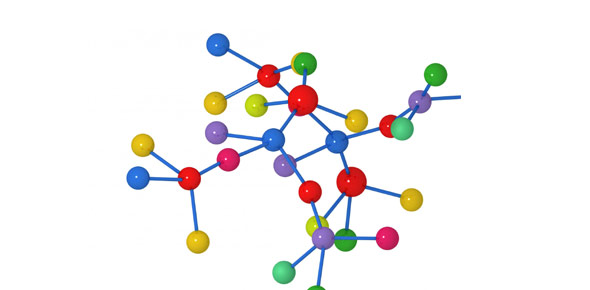Unit 3 Chee 370

25 Questions | Total Attempts: 41SettingsFrom the slides

• 1.
• A.

For long term storage of energy

• B.

High energy intermediates

• C.

Used once

• D.

Specific to eukaryotes

• 2.
ATP ----> ADP + Pi has dG=?
• A.

-3.06 kJ/mol

• B.

-30.6 kJ/mol

• C.

-300.6kJ/mol

• D.

300.6 kJ/mol

• 3.
What method of energy (ATP) production is anaerobic?
• 4.
What method of energy (ATP) production is aerobic?
• 5.
How many ATP is required in the preparatory phase of glycolysis to produce 2 GAP molecules?
• 6.
How many pyrvuvates, NADH, ATP do you get per GAP molecule in the energy production phase of glycolysis? (separate with commas, no spaces)
• 7.
What does fermentation produce?
• A.

• B.

• C.

• D.

• 8.
What are the inputs of acetyl coA production
• A.

• B.

• C.

Pyruvate

• D.

Coa

• 9.
How many NADH does Krebs (one cycle) produce?
• 10.
The other products of Krebs cycle (one cycle) are: 2 CO2, 2 GTP, 2 FADH, CoA
• A.

True

• B.

False

• 11.
During the ETC: FADH donates electrons to complex I, NADH donates electrons to complex II
• A.

True

• B.

False

• 12.
The electrons from complex I and II get shuttled off to ______ next
• A.

Complex III

• B.

Ubiquinone

• C.

Cytochrome C

• D.

Complex IIII

• 13.
Where does the electron transport chain occur in eukaryotes?
• A.

The cell membrane

• B.

Between the mitochondrial membranes (H+ pumped into intermembrane space)

• C.

The cytoplasm

• D.

The surface of the smooth endoplasmic reticulum

• 14.
Where does the electron transport chain occur in prokaryotes?
• A.

The cell membrane

• B.

Between the mitochondrial membranes (H+ pumped into intermembrane space)

• C.

The cytoplasm

• D.

On the surface of the smooth endoplasmic reticulum

• 15.
What is the enzyme that catalyses ADP + Pi ---> ATP
• 16.
How many ATP does each NADH produce (ETC)?
• 17.
How many ATP does FADH produce (ETC)?
• 18.
What's the efficiency of aerobic respiration?
• A.

25%

• B.

61.5%

• C.

41.5%

• D.

80%

• 19.
What's the total ATP yield for aerobic respiration?
• 20.
What are the phases of cell growth in culture in order?  ______ phase, _______ phase, ______ phase, ________phase (separate with commas, no spaces)
• 21.
If there are initially 2 cells, the generation time is 1 h/cell, and the culture has been in exponential phase for 12 hours, how many cells are there?
• 22.
As temperature decreases, enzymes function more efficiently
• A.

True

• B.

False

• 23.
An _______'s ideal environment is where ph<7
• 24.
Halophiles need high ______.
Related TopicsBack to top